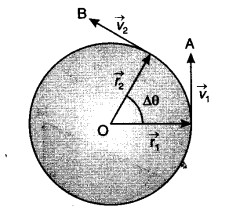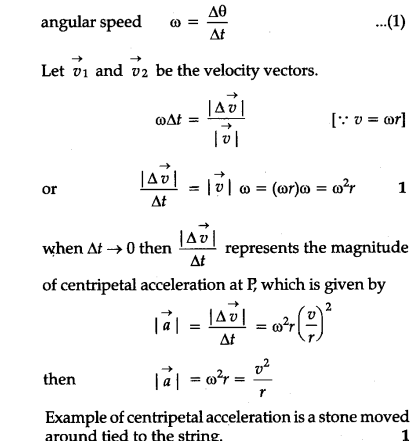# Define centripetal acceleration. Find the expression for it. Give one example of centripetal force

Acceleration acting on the object undergoing uniform circular motion is called centripetal acceleration.Expression : Consider a particle of mass m, moving with a constant speed v and uniform angular velocity \si , on a circular path of radius r with centre at O.1 Like